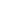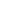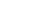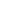xMagnetomotive forceEncyclopedia
Magnetomotive force (SI Unit: Ampere
Ampere
The ampere , often shortened to amp, is the SI unit of electric current and is one of the seven SI base units. It is named after André-Marie Ampère , French mathematician and physicist, considered the father of electrodynamics...

) is any physical driving (motive) force that produces magnetic flux
Magnetic flux
Magnetic flux , is a measure of the amount of magnetic B field passing through a given surface . The SI unit of magnetic flux is the weber...

. In this context, the expression "driving force" is used in a general sense of "work potential", and is analogous, but distinct from force
Force
In physics, a force is any influence that causes an object to undergo a change in speed, a change in direction, or a change in shape. In other words, a force is that which can cause an object with mass to change its velocity , i.e., to accelerate, or which can cause a flexible object to deform...

measured in newtons. The name came about because in magnetic circuit
Magnetic circuit
A magnetic circuit is made up of one or more closed loop paths containing a magnetic flux. The flux is usually generated by permanent magnets or electromagnets and confined to the path by magnetic cores consisting of ferromagnetic materials like iron, although there may be air gaps or other...

s it plays a role analogous to the role electromotive force
Electromotive force
In physics, electromotive force, emf , or electromotance refers to voltage generated by a battery or by the magnetic force according to Faraday's Law, which states that a time varying magnetic field will induce an electric current.It is important to note that the electromotive "force" is not a...

(voltage
Voltage
Voltage, otherwise known as electrical potential difference or electric tension is the difference in electric potential between two points — or the difference in electric potential energy per unit charge between two points...

) plays in electric circuits.

## SI versus CGS units

The SI unit of magnetomotive force is the ampere
Ampere
The ampere , often shortened to amp, is the SI unit of electric current and is one of the seven SI base units. It is named after André-Marie Ampère , French mathematician and physicist, considered the father of electrodynamics...

(A), represented by a steady, direct electric current
Electric current
Electric current is a flow of electric charge through a medium.This charge is typically carried by moving electrons in a conductor such as wire...

of one ampere
Ampere
The ampere , often shortened to amp, is the SI unit of electric current and is one of the seven SI base units. It is named after André-Marie Ampère , French mathematician and physicist, considered the father of electrodynamics...

flowing in a single-turn loop of electrically conducting material in a vacuum
Vacuum
In everyday usage, vacuum is a volume of space that is essentially empty of matter, such that its gaseous pressure is much less than atmospheric pressure. The word comes from the Latin term for "empty". A perfect vacuum would be one with no particles in it at all, which is impossible to achieve in...

.

The CGS unit of magnetomotive force is the gilbert (Gi), established by the IEC
International Electrotechnical Commission
The International Electrotechnical Commission is a non-profit, non-governmental international standards organization that prepares and publishes International Standards for all electrical, electronic and related technologies – collectively known as "electrotechnology"...

in 1930 http://www.iec.ch/about/history/. The gilbert is defined differently, and is a slightly smaller unit than the ampere. The unit is named after William Gilbert (1544–1603) English physician, astronomer and natural philosopher.

The conversion factor between the SI and CGS units is(≈ 0.795774715) ampere for every gilbert.

Between the CGS unit and SI unit, the MKS
Mks system of units
The MKS system of units is a physical system of units that expresses any given measurement using fundamental units of the metre, kilogram, and/or second ....

unit magnetomotive force was the ampere-turn At
Ampere-turn
The ampere-turn was the MKS unit of magnetomotive force , represented by a direct current of one ampere flowing in a single-turn loop in a vacuum...

.

## Equations

The magnetomotive forcein an inductor
Inductor
An inductor is a passive two-terminal electrical component used to store energy in a magnetic field. An inductor's ability to store magnetic energy is measured by its inductance, in units of henries...

or electromagnet
Electromagnet
An electromagnet is a type of magnet in which the magnetic field is produced by the flow of electric current. The magnetic field disappears when the current is turned off...

consisting of a coil of wire is given by:where N is the number of turns of wire in the coil and I is the current in the wire.

The equation for the magnetic flux in a magnetic circuit, sometimes known as Hopkinson's law, is:where Φ is the magnetic flux andis the reluctance of the magnetic circuit. It can be seen that the magnetomotive force plays a role in this equation analogous to the voltage V in Ohm's law
Ohm's law
Ohm's law states that the current through a conductor between two points is directly proportional to the potential difference across the two points...

: V = IR.